# Boosting, Bagging, and Stacking — Ensemble Methods with sklearn and mlens

Binning, bagging, and stacking, are basic parts of a data scientist’s toolkit and a part of a series of statistical techniques called ensemble methods. The GitHub for this project can be found here.Decisions …

There are three main terms describing the ensemble (combination) of various models into one more effective model:

• Bagging to decrease the model’s variance;
• Boosting to decreasing the model’s bias, and;
• Stacking to increasing the predictive force of the classifier.

What is an ensemble method?

The idea here is to train multiple models, each with the objective to predict or classify a set of results.

Most of the errors from a model’s learning are from three main factors: variance, noise, and bias. By using ensemble methods, we’re able to increase the stability of the final model and reduce the errors mentioned previously. By combining many models, we’re able to (mostly) reduce the variance, even when they are individually not great, as we won’t suffer from random errors from a single source.

The main principle behind ensemble modelling is to group weak learners together to form one strong learner.

From many, together they emerge as one.

Consider — as I’ll show below — housing prices and how you would think about classifying a person’s house selling price as low, medium, or high. Is there one rule you would use to determine this classification, or would you use a variety of rules based on different factors?

You would of course use various rules, such as:

• Walking distance to public schools;
• The age of the house and expected renovations;
• How big the backyard is;
• Drive time to the city and the accessibility of transit, etc.

Just as these few questions cannot be solved by one analysis, but rather solved by different and overlapping analytical approaches. And, this is the heart of ensemble modelling.

The Housing Dataset

The example below looks at housing data, to see if the price classification of a house (low, medium, and high) can be determined by a few characteristics:

• The lot size;
• No. of bedrooms;
• No. of bathrooms;
• No. of stories;
• Has driveway, etc.

The housing data from `pydataset` was used to show the difference in classification accuracy for our various ensemble methods. As a caveat, I think this is a good dataset, however there are too many binary variables. If I was to go back and redo this project, I would possibly use the Boston housing dataset which has more features and flexibility for geolocation feature engineering.

Let’s take a look at our data.

`from pydataset import data`
`# Get the housing datadf = data('Housing')df.head().values`
`# Check the dataarray([[42000.0, 5850, 3, 1, 2, 'yes', 'no', 'yes', 'no', 'no', 1, 'no'],       [38500.0, 4000, 2, 1, 1, 'yes', 'no', 'no', 'no', 'no', 0, 'no'],       [49500.0, 3060, 3, 1, 1, 'yes', 'no', 'no', 'no', 'no', 0, 'no'], ...`
`# Create dictionary to label 'yes' and 'no'd = dict(zip(['no', 'yes'], range(0,2)))`
`for i in zip(df.dtypes.index, df.dtypes):    if str(i) == 'object':        df[i] = df[i].map(d)`

Before we bucket our prices, let’s look at the ranges of our prices.

`for i, j in enumerate(np.unique(pd.qcut(df['price'], 3))):    print i, j`
`# Results0 (24999.999, 53000.0]1 (53000.0, 74500.0]2 (74500.0, 190000.0]`

Looks like the lowest house we have in the dataset is \$25K, and the highest is \$190K. Not the most expensive houses on the market, but it will do for the examples below. Now let’s bucket the prices.

`df[‘price’] = pd.qcut(df[‘price’], 3, labels=[‘0’, ‘1’, ‘2’]).cat.codes`
`# Split into two setsy = df['price'] X = df.drop('price', 1)`

Now we have something to work with. It’s not fancy, and it should be enough to illustrate the point about about the power of ensemble models.

There is not going to any data exploration with this article, but let’s look at a quick correlation plot to see what we’re working with for our housing data.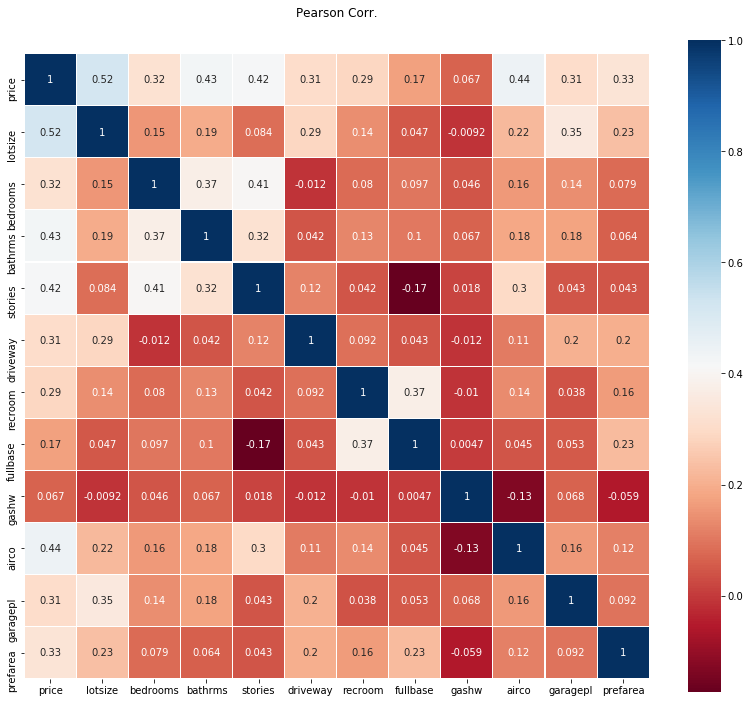Correlation plot for our housing data

Nothing too interesting here, except it looks as the number of stories of a house increases the chance there is a basement decreases. The same is true with driveways and bedrooms, but only slightly.

And for completeness, here is a pairwise plot of some of the more interesting relationships shown from the heat-map above.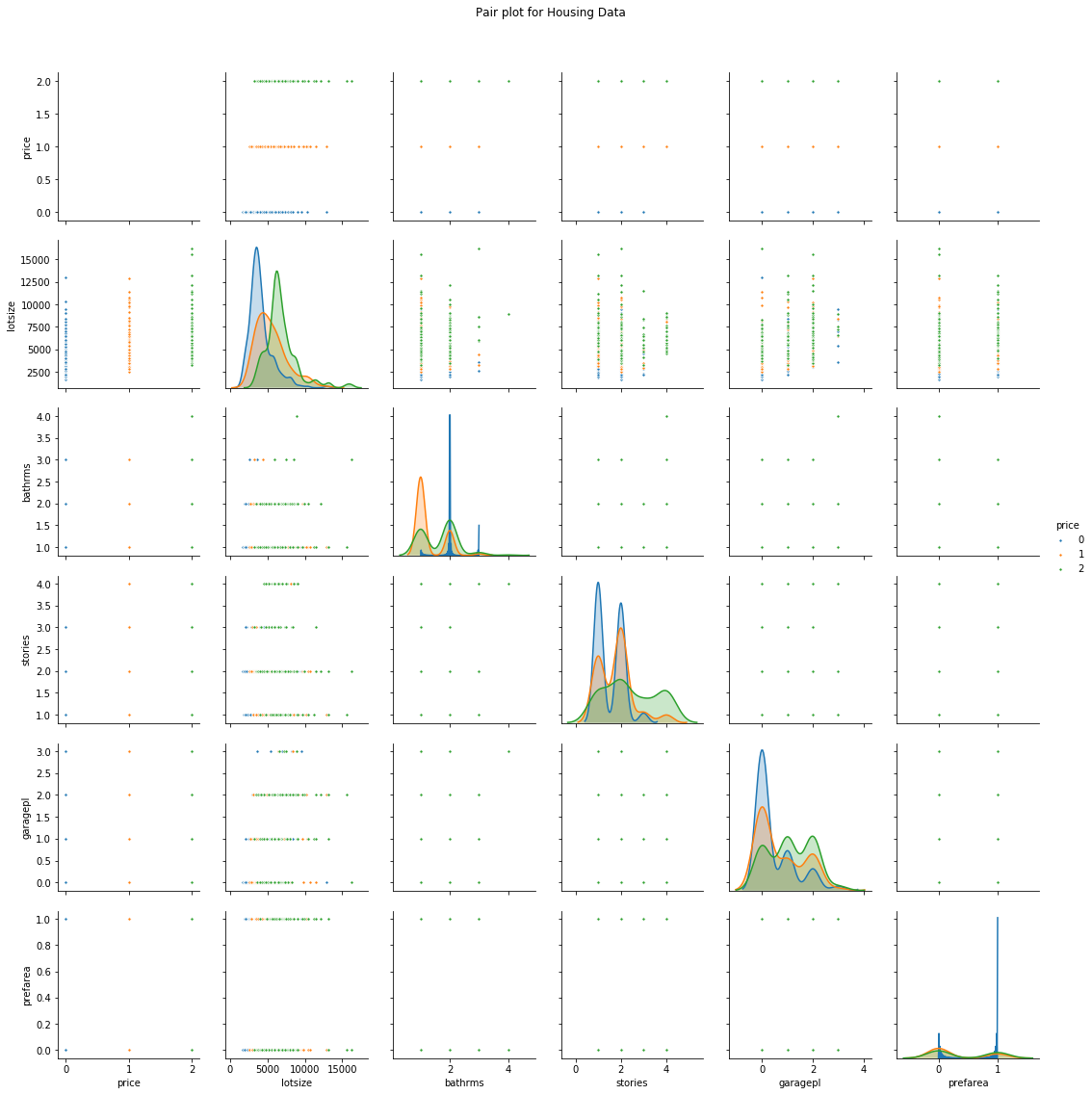Pairwise plot of selected parts of our data

Starting with Bagging

The first term introduced, bagging, is shorthand for the combination of bootstrapping and aggregating. Bootstrapping is a method to help decrease the variance of the classifier and reduce overfitting, by resampling data from the training set with the same cardinality as the original set. The model created should be less overfitted than a single individual model.

A high variance for a model is not good, suggesting its performance is sensitive to the training data provided. So, even if more the training data is provided, the model may still perform poorly. And, may not even reduce the variance of our model.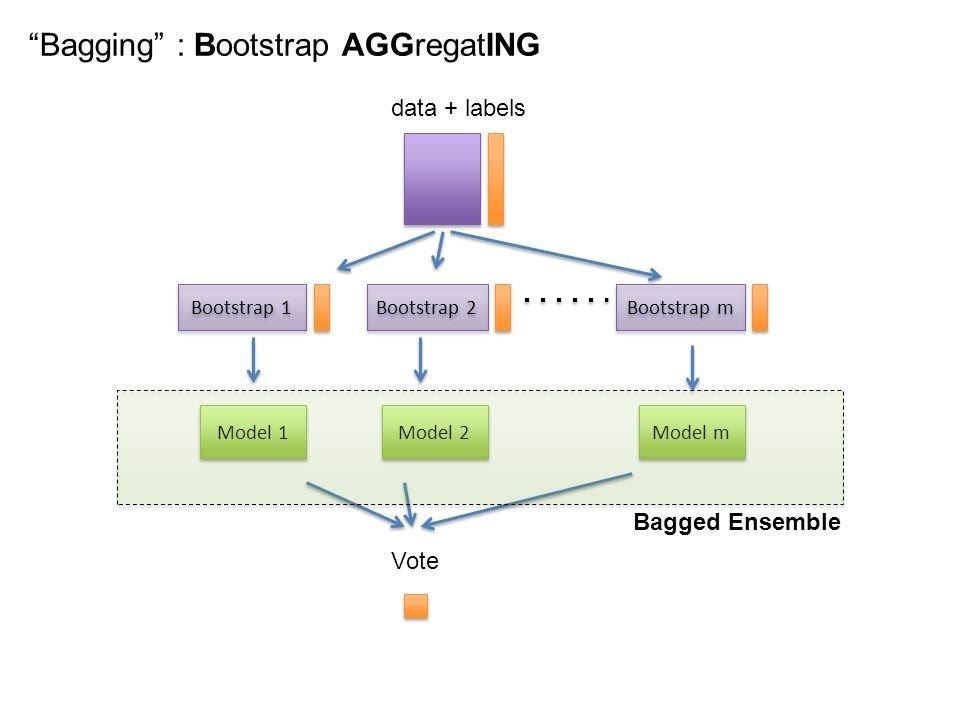Easy visualization of what Bagging does; each model is individual and voted upon

Bagging is an effective method when you have limited data, and by using samples you’re able to get an estimate by aggregating the scores over many samples.

The simplest approach with bagging is to use a couple of small subsamples and bag them, if the ensemble accuracy is much higher than the base models, it’s working; if not, use larger subsamples.

`# Bagging RandomForestClassifier at different subsamples`
`# Bagging classifier at .1 subsamplesAvg. Accuracy of: 0.641 (+/-) 0.080`
`# Bagging classifier at .3 subsamplesAvg. Accuracy of: 0.650 (+/-) 0.091     # Better accuracy`
`# Bagging classifier at .5 subsamplesAvg. Accuracy of: 0.639 (+/-) 0.091     # Worse accuracy`

Note that using larger subsamples is not guaranteed to improve your results. In bagging there is a tradeoff between base model accuracy and the gain you get through bagging. The aggregation from bagging may improve the ensemble greatly when you have an unstable model, yet when your base models are more stable — been trained on larger subsamples with higher accuracy — improvements from bagging reduces.

Once the bagging is done, and all the models have been created on (mostly) different data, a weighted average is then used to determine the final score.

`# Get some classifiers to evaluatefrom sklearn.model_selection import cross_val_scorefrom sklearn.ensemble import BaggingClassifier, ExtraTreesClassifier, RandomForestClassifierfrom sklearn.neighbors import KNeighborsClassifierfrom sklearn.linear_model import RidgeClassifierfrom sklearn.svm import SVC`
`seed = 1075np.random.seed(seed)`
`# Create classifiersrf = RandomForestClassifier()et = ExtraTreesClassifier()knn = KNeighborsClassifier()svc = SVC()rg = RidgeClassifier()`
`clf_array = [rf, et, knn, svc, rg]`
`for clf in clf_array:    vanilla_scores = cross_val_score(clf, X, y, cv=10, n_jobs=-1)    bagging_clf = BaggingClassifier(clf,        max_samples=0.4, max_features=10, random_state=seed)    bagging_scores = cross_val_score(bagging_clf, X, y, cv=10,        n_jobs=-1)        print "Mean of: {1:.3f}, std: (+/-) {2:.3f [{0}]"                         .format(clf.__class__.__name__,                        vanilla_scores.mean(), vanilla_scores.std())    print "Mean of: {1:.3f}, std: (+/-) {2:.3f} [Bagging {0}]\n"                       .format(clf.__class__.__name__,                         bagging_scores.mean(), bagging_scores.std())`

How did our bagging do against version the vanilla versions of our classifiers?

`Mean of: 0.632, std: (+/-) 0.081 [RandomForestClassifier]Mean of: 0.639, std: (+/-) 0.069 [Bagging RandomForestClassifier]Mean of: 0.636, std: (+/-) 0.080 [ExtraTreesClassifier]Mean of: 0.654, std: (+/-) 0.073 [Bagging ExtraTreesClassifier]Mean of: 0.500, std: (+/-) 0.086 [KNeighborsClassifier]Mean of: 0.535, std: (+/-) 0.111 [Bagging KNeighborsClassifier]Mean of: 0.465, std: (+/-) 0.085 [SVC]Mean of: 0.535, std: (+/-) 0.083 [Bagging SVC]Mean of: 0.639, std: (+/-) 0.050 [RidgeClassifier]Mean of: 0.597, std: (+/-) 0.045 [Bagging RidgeClassifier]`

In all but one of the classifiers, we had lower variance. As well, the accuracy of our classifiers all increased except for our ridge classifier. Looks like this bagging thing actually works. Who would have thought?

So our bagged individual classifiers are (mostly) better, but which one do we choose?

Let’s Vote! — How Do You Voting?

Sklearn’s `VotingClassifier` allows you to combine different machine learning classifiers, and perform a vote on what the predicted class label(s) are for a record.

There are two types of voting you can do for classifiers: hard and soft.

With hard voting, you just need a majority of classifiers to determine what the result could be. As with the image below, the various bagged models are shown with `H`, and the results of the classifiers are shown on the rows. On the far right, `H1` and `H3` vote for the first record to be “no” (purple) while `H2` votes for “yes” (yellow). Because 2 of the models vote for “no”, the ensemble classifies that record as a “no”.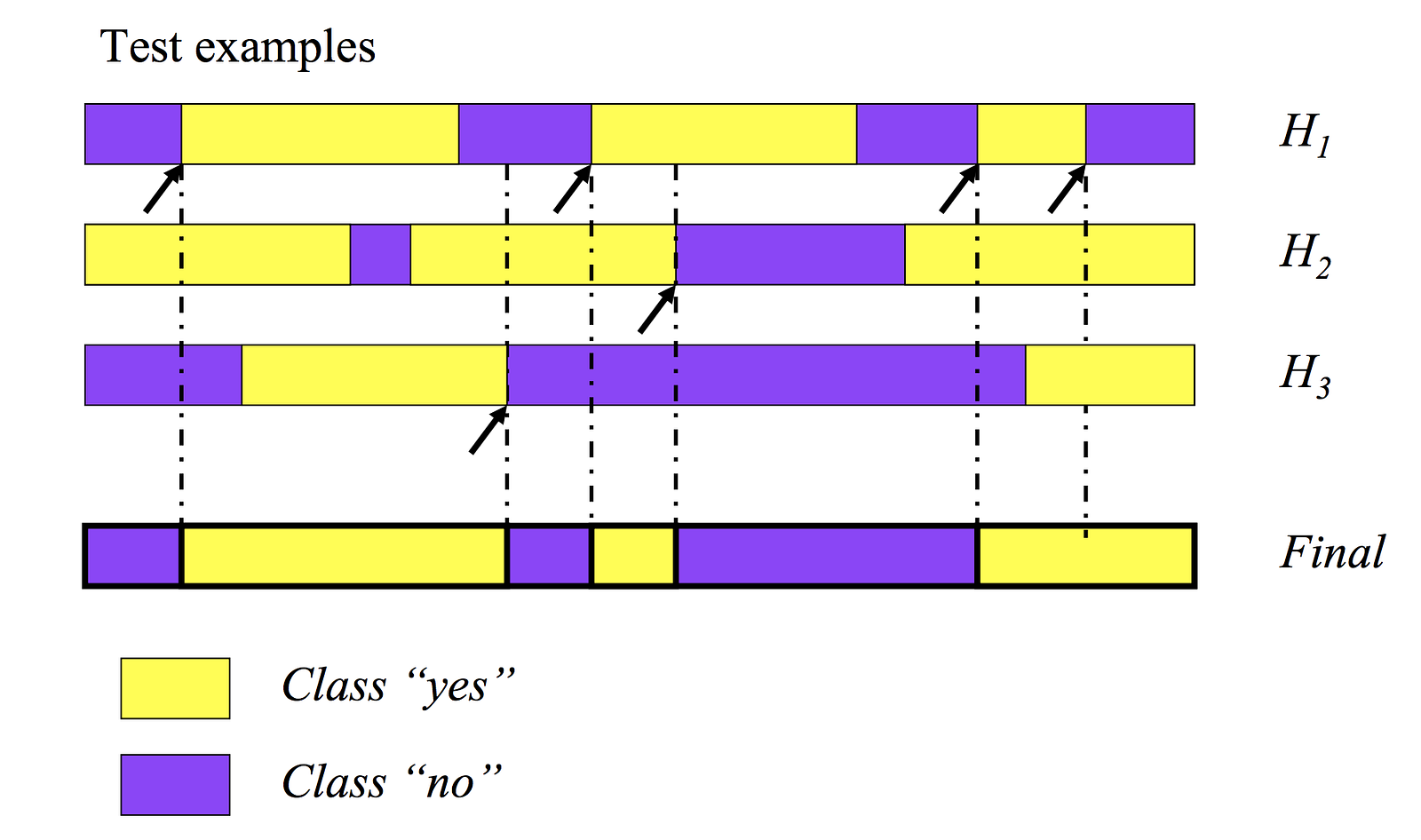A visual example of how hard (majority) voting works.

With soft (weighted), we compute a percentage weight with each classifier. A predicted class probability from each model for each record is collected and multiplied by the classifier weight, and finally averaged. The final class label is then derived from the class label with the highest average probability.

In reality weights are hard to find if you’re just providing your best guesses to which model you think should be weighted more or less. To counter this subjective process, a linear optimization equation or neural net could be constructed to find the correct weighting for each of the models to optimize the accuracy of the ensemble.

`# Example of hard voting from sklearn.ensemble import VotingClassifier`
`clf = [rf, et, knn, svc, rg]eclf = VotingClassifier(estimators=[('Random Forests', rf), ('Extra Trees', et), ('KNeighbors', knn), ('SVC', svc), ('Ridge Classifier', rg)], voting='hard')`
`for clf, label in zip([rf, et, knn, svc, rg, eclf], ['Random Forest', 'Extra Trees', 'KNeighbors', 'SVC', 'Ridge Classifier', 'Ensemble']):    scores = cross_val_score(clf, X, y, cv=10, scoring='accuracy')    print("Accuracy: %0.2f (+/- %0.2f) [%s]" % (scores.mean(), scores.std(), label))`
`# ResultsMean: 0.627, std: (+/-) 0.082 [Random Forest]Mean: 0.632, std: (+/-) 0.084 [Extra Trees]Mean: 0.500, std: (+/-) 0.086 [KNeighbors]Mean: 0.465, std: (+/-) 0.085 [SVC]Mean: 0.639, std: (+/-) 0.050 [Ridge Classifier]Mean: 0.641, std: (+/-) 0.094 [Ensemble]`
`# Compare this to the bagged resultsMean: 0.661, std: (+/-) 0.099 [Bagging Random Forest]Mean: 0.650, std: (+/-) 0.082 [Bagging Extra Trees]Mean: 0.535, std: (+/-) 0.117 [Bagging KNeighbors]Mean: 0.528, std: (+/-) 0.068 [Bagging SVC]Mean: 0.604, std: (+/-) 0.046 [Bagging Ridge Classifier]Mean: 0.658, std: (+/-) 0.091 [Bagging Ensemble]`

With our bagged ensemble results, we have an increase in accuracy(`.658` against `.641`) and a decrease in variance (`.091` against `.094`), so our ensemble model is working as expected after we’ve combined all the various models into one.

Decision Boundaries

Now that we know how well our model(s) are doing individually and together, does that actually look. How can we see the boundaries of our classifiers and determine where their cut off points are?

Luckily `mlxtend` has a great `plot_decision_regions` which show how each classifier make its decisions. Because there are more than two features that go into the decision process for each classification, the plot below only shows the decision boundaries for `lotsize` and `stories`.

We’re going to use the `eclf` classifier we defined in the previous voting phase of the project, and use the results of the classifications to compare itself to the vanilla models we made earlier.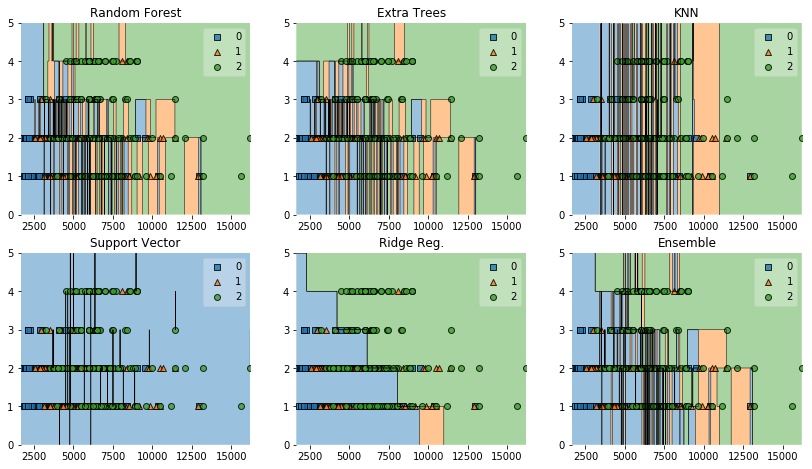plot_decision_regions on each of our classifiers for lotsize and stores

Here is how you can recreate the graph.

`import matplotlib.pyplot as pltfrom mlxtend.plotting import plot_decision_regionsimport matplotlib.gridspec as gridspecimport itertools`
`gs = gridspec.GridSpec(3, 3)`
`fig = plt.figure(figsize=(14, 12))`
`labels = ['Random Forest', 'Extra Trees', 'KNN', 'Support Vector',          'Ridge Reg.', 'Ensemble']for clf, lab, grd in zip([rf, et, knn, svc, rg, eclf],                          labels,                         itertools.product([0, 1, 2], repeat = 2)):`
`clf.fit(X[['lotsize', 'stories']], y)    ax = plt.subplot(gs[grd, grd])    fig = plot_decision_regions(X=np.array(X[['lotsize', 'stories']]),                                 y=np.array(y), clf=clf)    plt.title(lab)`

Boosting

Think about optimization of a function over its function space, where optimization can be solved using gradient descent. Vanilla gradient gradient descent is used to minimize a set of parameters. E.g. finding the weights of parameters for a linear regression, through updates from an error function.

Parameter estimation seems trivial if we have a smooth convex parameter space, however not all problems provide such a simple plane to traverse over. Our problem, because there many categorial and binary variables it creates a complex gradient with many local minima to get stuck in during the optimization process. For these problems, we can use a different form of gradient descent called boosting.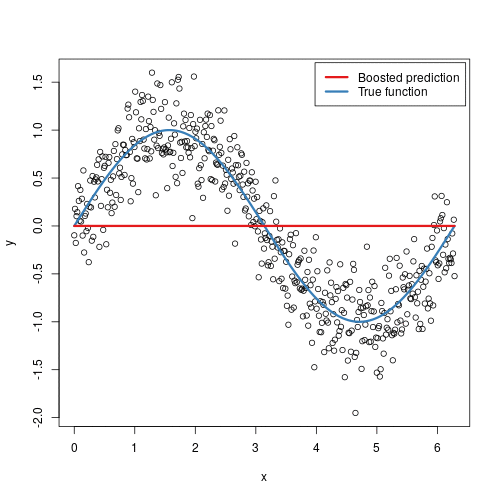How boosting looks; from (github.com/bgreenwell/)

The main idea of boosting is to add additional models to the overall ensemble model sequentially. Previously with bagging, we averaged each individual model created. This time with each iteration of boosting, a new model is created and the new base-learner model is trained (updated) from the errors of the previous learners.

The algorithm creates multiple weak models whose output is added together to get an overall prediction. This is ensemble modelling from earlier. The now boosted gradient shifts the current prediction nudging it to the true target, in a similar fashion to how gradient descent moves toward the true values. The gradient descent optimization occurs on the output of the varies models, and not their individual parameters.

There are different methods to optimize boosting algorithms, but they are beyond the scope of this article.

Unlike the bagging examples above, classical boosting the subset creation is not random and performance will depend upon the performance of previous models. As, each new subset which is iterated upon contains elements which could have been misclassified by previous models. We will also be using the same hard voting we used previously to ensemble the models together.

`ada_boost = AdaBoostClassifier()grad_boost = GradientBoostingClassifier()xgb_boost = XGBClassifier()`
`boost_array = [ada_boost, grad_boost, xgb_boost]`
`eclf = EnsembleVoteClassifier(clfs=[ada_boost, grad_boost, xgb_boost], voting='hard')`
`labels = ['Ada Boost', 'Grad Boost', 'XG Boost', 'Ensemble']`
`for clf, label in zip([ada_boost, grad_boost, xgb_boost, eclf], labels):    scores = cross_val_score(clf, X, y, cv=10, scoring='accuracy')    print("Mean: {0:.3f}, std: (+/-) {1:.3f} [{2}]".format(scores.mean(), scores.std(), label))Let’s perform the same of voting on our boosting models`
`# Results`
`Mean: 0.641, std: (+/-) 0.082 [Ada Boost]Mean: 0.654, std: (+/-) 0.113 [Grad Boost]Mean: 0.663, std: (+/-) 0.101 [XG Boost]Mean: 0.667, std: (+/-) 0.105 [Ensemble]`

And, how do the decision boundaries look for the boosting algorithms? Interestingly enough the ensemble for both the boosted and the bagged are quite similar.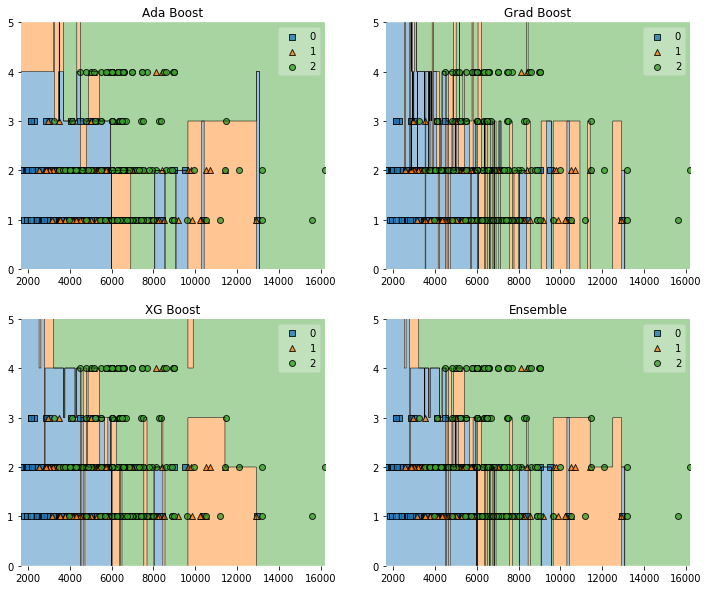Boosting algorithms decision boundaries with an ensemble

Overall it seems our ensemble model for boosting provided an accuracy, `0.667 `which was just over the bagging’s ensemble’s score`0.658`. This is not saying boosting is better than bagging, as in these examples nothing has been optimized. It should also be noted the standard deviation of the boosted model is higher, which is to be expected.

However, there is one more ensemble method to look at.

Stacking

Stacking is another ensemble model, where a new model is trained from the combined predictions of two (or more) previous model. The predictions from the models are used as inputs for each sequential layer, and combined to form a new set of predictions. These can be used on additional layers, or the process can stop here with a final result. In the example below I’m only going to use one layer for simplicity.

Ensemble stacking can be referred to as blending, because all the numbers are blended to produce a prediction or classification.

Keep in mind just by adding layers and more models to your stacking algorithm, does not mean you’ll get a better predictor. There are no free lunches in machine learning.

E.g. with my stacking model I removed `SVM` from the layers, and ended up increasing the accuracy from `.52` to `.68`. And, this will become more clear in a moment.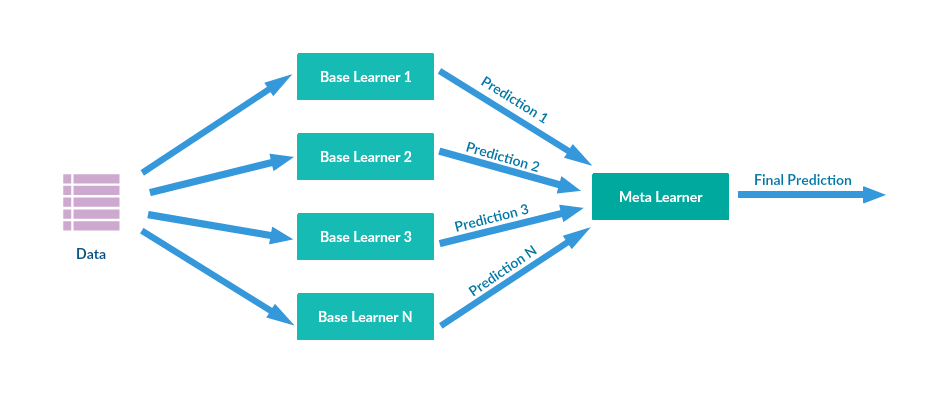Stacking visualized; Image from http://supunsetunga.blogspot.com/

Normally creating a stacking pipeline would be … difficult. However there is a great package ML Ensemble which simplifies the process, and allows you to focus on the work and optimizing the model, rather than simply the coding.

I’ve taken the vanilla classifiers `[‘Random Forest’, ‘Extra Trees’, ‘KNeighbors’, ‘SVC’, ‘Ridge Classifier’]` we started with, and combined them into all possible combinations to test which will perform best in our stacked model.

E.g. [‘SVC’, ‘Ridge Classifier’], [‘SVC’], [‘Random Forest’, ‘Extra Trees’, ‘KNeighbors’], etc.

Once this is complete, we should have something to compare to our previous two results. `Mlens` does come packaged with a model selector, however I wanted to show the process manually.

Finally we’ll be using a logistic regression as the final output layer, as we still want to classify out housing data.

`from itertools import combinationsfrom sklearn.linear_model import LogisticRegression`
`lr = LogisticRegression()`
`names = ['Random Forest', 'Extra Trees', 'KNeighbors', 'SVC', 'Ridge Classifier']`
`def zip_stacked_classifiers(*args):    to_zip = []    for arg in args:        combined_items = sum([map(list, combinations(arg, i)) for i in range(len(arg) + 1)], [])        combined_items = filter(lambda x: len(x) > 0, combined_items)        to_zip.append(combined_items)        return zip(to_zip, to_zip)`
`stacked_clf_list = zip_stacked_classifiers(clf_array, names)`
`best_combination = [0.00, ""]`
`for clf in stacked_clf_list:        ensemble = SuperLearner(scorer = accuracy_score,                             random_state = seed,                             folds = 10)    ensemble.add(clf)    ensemble.add_meta(lr)    ensemble.fit(X_train, y_train)    preds = ensemble.predict(X_test)    accuracy = accuracy_score(preds, y_test)        if accuracy > best_combination:        best_combination = accuracy        best_combination = clf        print("Accuracy score: {:.3f} {}").format(accuracy, clf)`
`print("\nBest stacking model is {} with accuracy of: {:.3f}").format(best_combination, best_combination)`
`# OutputAccuracy score: 0.674 ['Random Forest']Accuracy score: 0.663 ['Extra Trees']Accuracy score: 0.547 ['KNeighbors']Accuracy score: 0.481 ['SVC']...`
`Best stacking model is ['Extra Trees', 'KNeighbors', 'SVC'] with accuracy of: 0.691`

Looks like we’ve beaten both the boosting and bagging model with a score of `.691`. Nice.

While this example just had one layer, the magic of `mlens` is we’re able to stack more and more layers quite easily.

Additionally you’re able to do a pseudo grid-search on the parameter space for each model, using the `Evaluator` package to find the optimal values for each model you’re using in the stack. What I’ve done is just a trivial implementation of that process, and there are further optimizations which could occur.

Final Thoughts

Even though `.691` is a fairly weak classifier, I hope the processes of going through each stage of ensemble modelling and voting was helpful. As with most of data science, the bulk of the work will go into testing and tuning hyperparameters, as well as feature engineering to improve the model.

The main point of the article was to show ensemble modelling can produce better predictions, and the various methods used to evaluate the models created.

As always, I hope you found this article helpful and learned something new.

Additional Reading